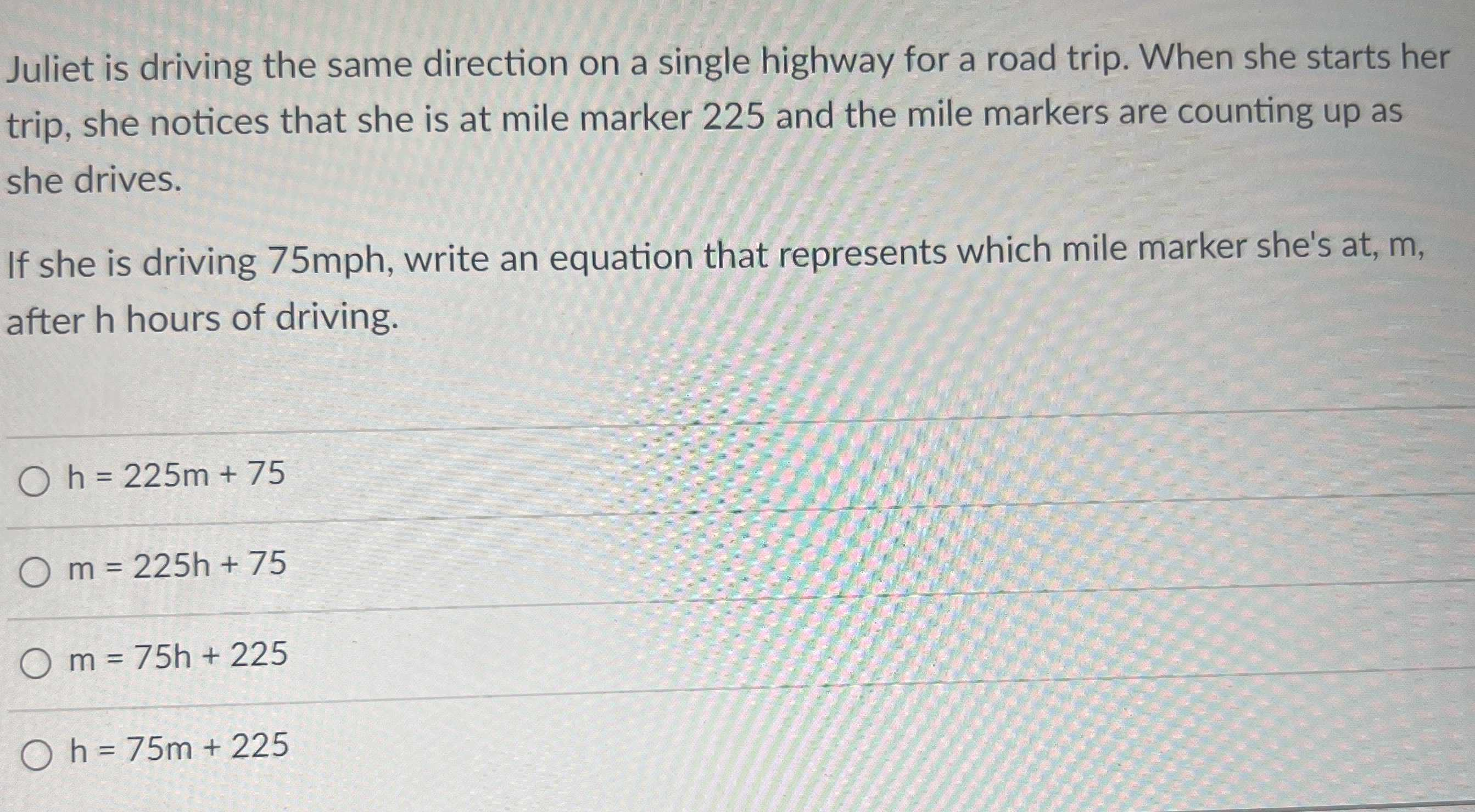### Still have math questions?

Algebra
QuestionJuliet is driving the same direction on a single highway for a road trip. When she starts her trip, she notices that she is at mile marker $$225$$ and the mile markers are counting up as she drives.

If she is driving $$75$$ mph, write an equation that represents which mile marker she's at, $$m$$ , after h hours of driving.

$$h = 225 m + 75$$

$$m = 225 h + 75$$

$$m = 75 h + 225$$

$$h = 75 m + 225$$